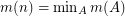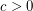# Chowla's cosine problem

 Importance: High ✭✭✭
 Subject: Number Theory
 Keywords: circle cosine polynomial
 Posted by: mdevos on: February 22nd, 2008
Problem   Letbe a set ofpositive integers and setWhat is?

It is easy to see that, since the average value of the sum of the cosines is zero. Bourgain [B] proved thatfor someandsufficiently large. Recently, Ruzsa [R] tightened this argument, proving thatwhere. The proof utilizes a clever manipulation of norms to reveal a (somewhat surprising) additive structure to the problem.

It seems the only known upper bound is.

## Bibliography

[B] J. Bourgain, Sur le minimum d'une somme de cosinus, Acta Arith. 45 (1986), 381--389. MathSciNet

*[C] S. Chowla, Some applications of a method of A. Selberg. J. Reine Angew. Math. 217 (1965) 128--132. MathSciNet

[R] I.Z. Ruzsa, Negative values of cosine sums. Acta Arith. 111 (2004), no. 2, 179--186. MathSciNet

* indicates original appearance(s) of problem.